# 1 Preparing the data

To demonstrate the data visualization of a `QFeatures`, we first perform a quick processing of the `hlpsms` example data. We load the data and read it as a `QFeautres` object. See the processing vignette for more details about data processing with `QFeatures`.

``````library("QFeatures")
data(hlpsms)
hl <- readQFeatures(hlpsms, ecol = 1:10, name = "psms")``````

We then aggregate the psms to peptides, and the peptodes to proteins.

``hl <- aggregateFeatures(hl, "psms", "Sequence", name = "peptides", fun = colMeans)``
``````## Your row data contain missing values. Please read the relevant
## section(s) in the aggregateFeatures manual page regarding the effects
## of missing values on data aggregation.``````
``hl <- aggregateFeatures(hl, "peptides", "ProteinGroupAccessions", name = "proteins", fun = colMeans)``

We also add the TMT tags that were used to multiplex the samples. The data is added to the `colData` of the `QFeatures` object and will allow us to demonstrate how to plot data from the `colData`.

``````hl\$tag <- c("126", "127N", "127C", "128N", "128C", "129N", "129C",
"130N", "130C", "131")``````

The dataset is now ready for data exploration.

# 2 Exploring the `QFeatures` hierarchy

`QFeatures` objects can contain several assays as the data goes through the processing workflow. The `plot` function provides an overview of all the assays present in the dataset, showing also the hierarchical relationships between the assays as determined by the `AssayLinks`.

``plot(hl)``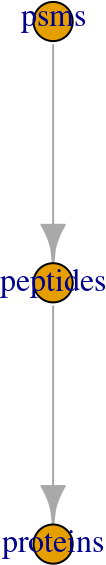This plot is rather simple with only three assays, but some processing workflows may involve more steps. The `feat3` example data illustrates the different possible relationships: one parent to one child, multiple parents to one child and one parent to multiple children.

``````data("feat3")
plot(feat3)``````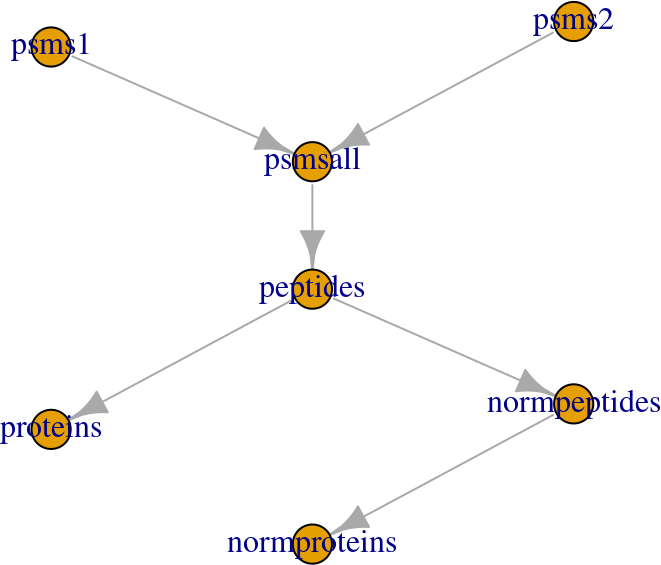Note that some datasets may contain many assays, for instance because the MS experiment consists of hundreds of batches. This can lead to an overcrowded plot. Therefore, you can also explore this hierarchy of assays through an interactive plot, supported by the `plotly` package (Sievert (2020)). You can use the viewer panel to zoom in and out and navigate across the tree(s).

``plot(hl, interactive = TRUE)``

# 3 Basic data exploration

The quantitative data is retrieved using `assay()`, the feature metadata is retrieved using `rowData()` on the assay of interest, and the sample metadata is retrieved using `colData()`. Once retrieved, the data can be supplied to the base R data exploration tools. Here are some examples:

• Plot the intensities for the first protein. These data are available from the `proteins` assay.
``plot(assay(hl, "proteins")[1, ])``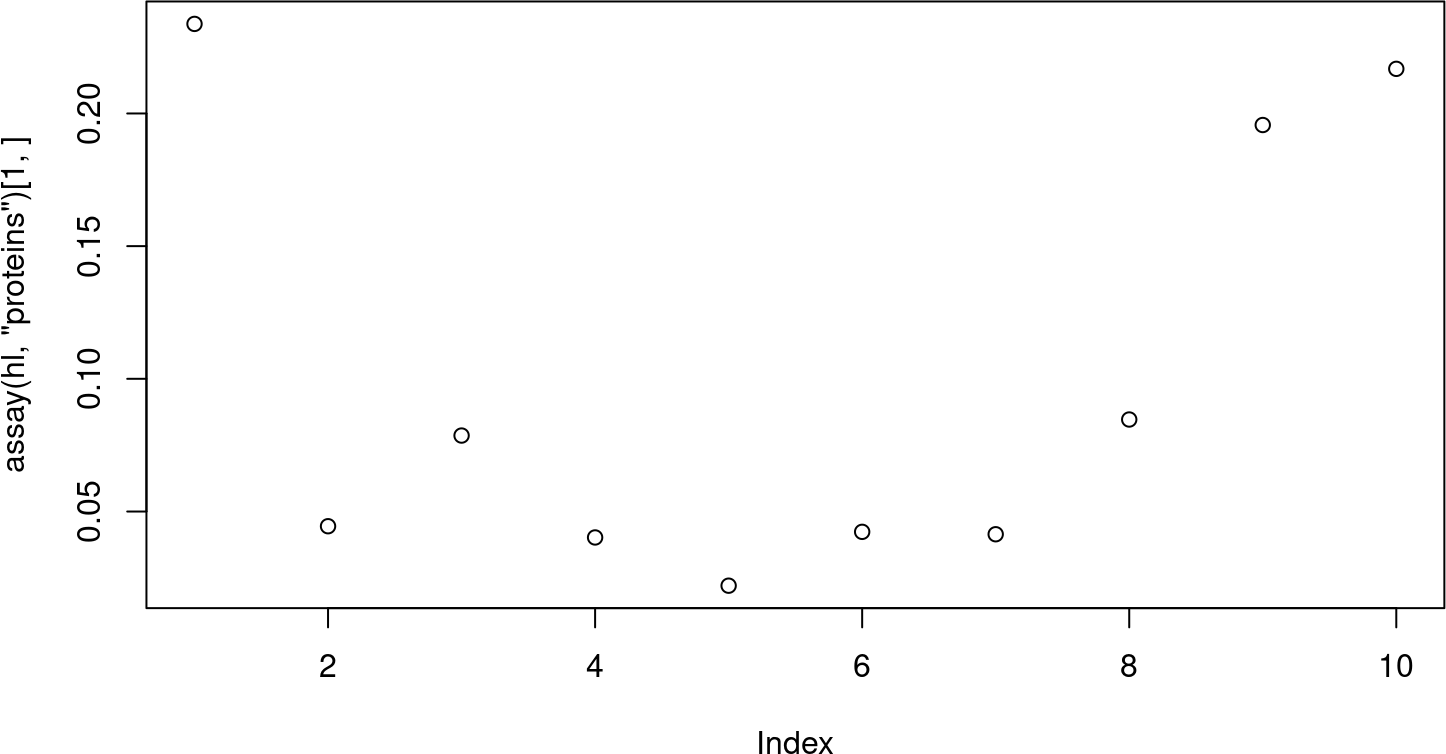• Get the distribution of the number of peptides that were aggregated per protein. These data are available in the column `.n` from the protein `rowData`.
``hist(rowData(hl)[["proteins"]]\$.n)``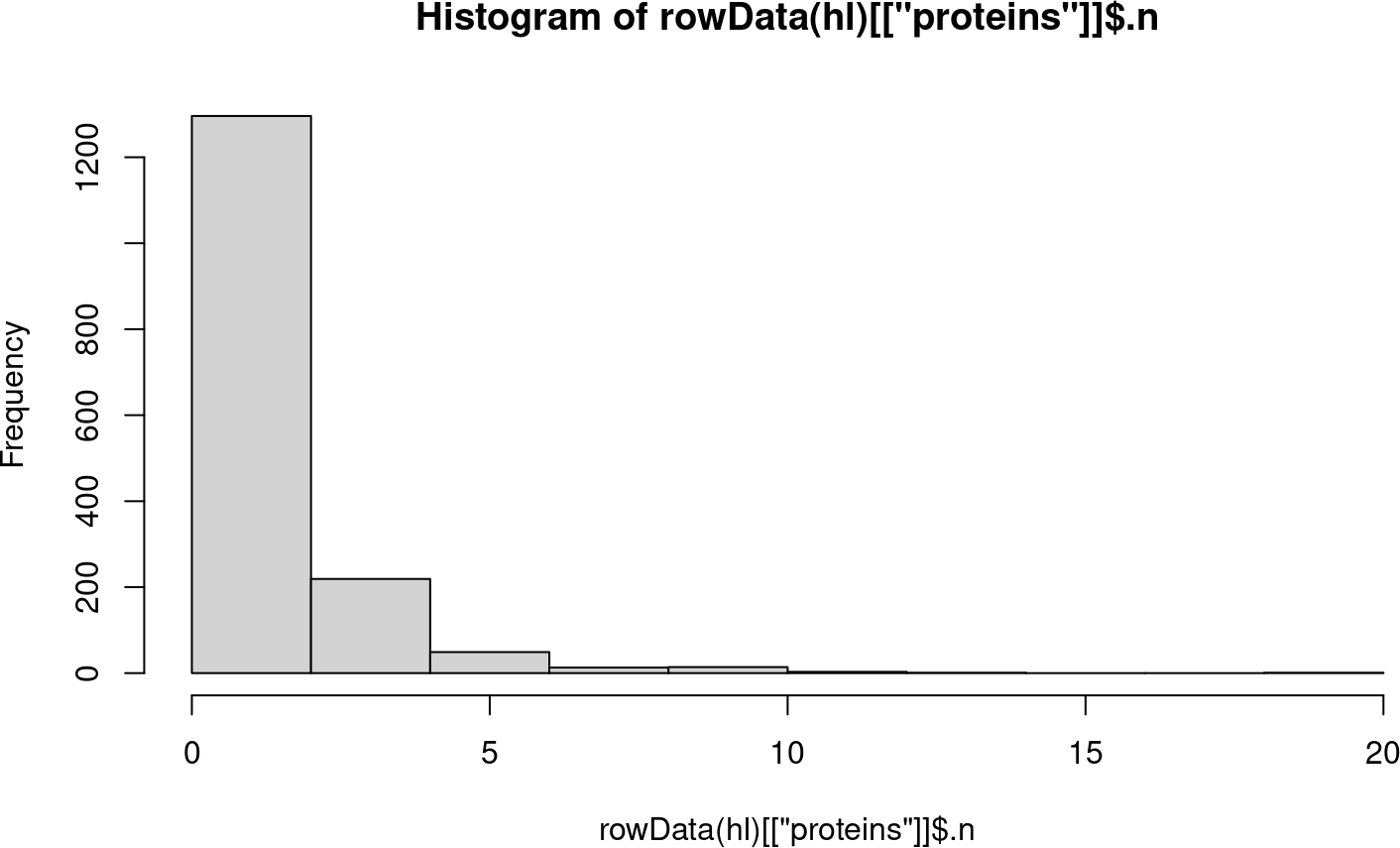• Get the count table of the different tags used for labeling the samples. These data are available in the column `tag` from the `colData`.
``table(hl\$tag)``
``````##
##  126 127C 127N 128C 128N 129C 129N 130C 130N  131
##    1    1    1    1    1    1    1    1    1    1``````

# 4 Using `ggplot2`

`ggplot2` is a powerful tool for data visualization in `R` and is part of the `tidyverse` package ecosystem (Wickham et al. (2019)). It produces elegant and publication-ready plots in a few lines of code. `ggplot2` can be used to explore `QFeatures` object, similarly to the base functions shown above. Note that `ggplot2` expects `data.frame` or `tibble` objects whereas the quantitative data in `QFeatures` are encoded as `matrix` (or matrix-like objects, see `?SummarizedExperiment`) and the `rowData` and `colData` are encoded as `DataFrame`. This is easily circumvented by converting those objects to `data.frame`s or `tibble`s. See here how we reproduce the plot above using `ggplot2`.

``````library("ggplot2")
df <- data.frame(rowData(hl)[["proteins"]])
ggplot(df) +
aes(x = .n) +
geom_histogram()``````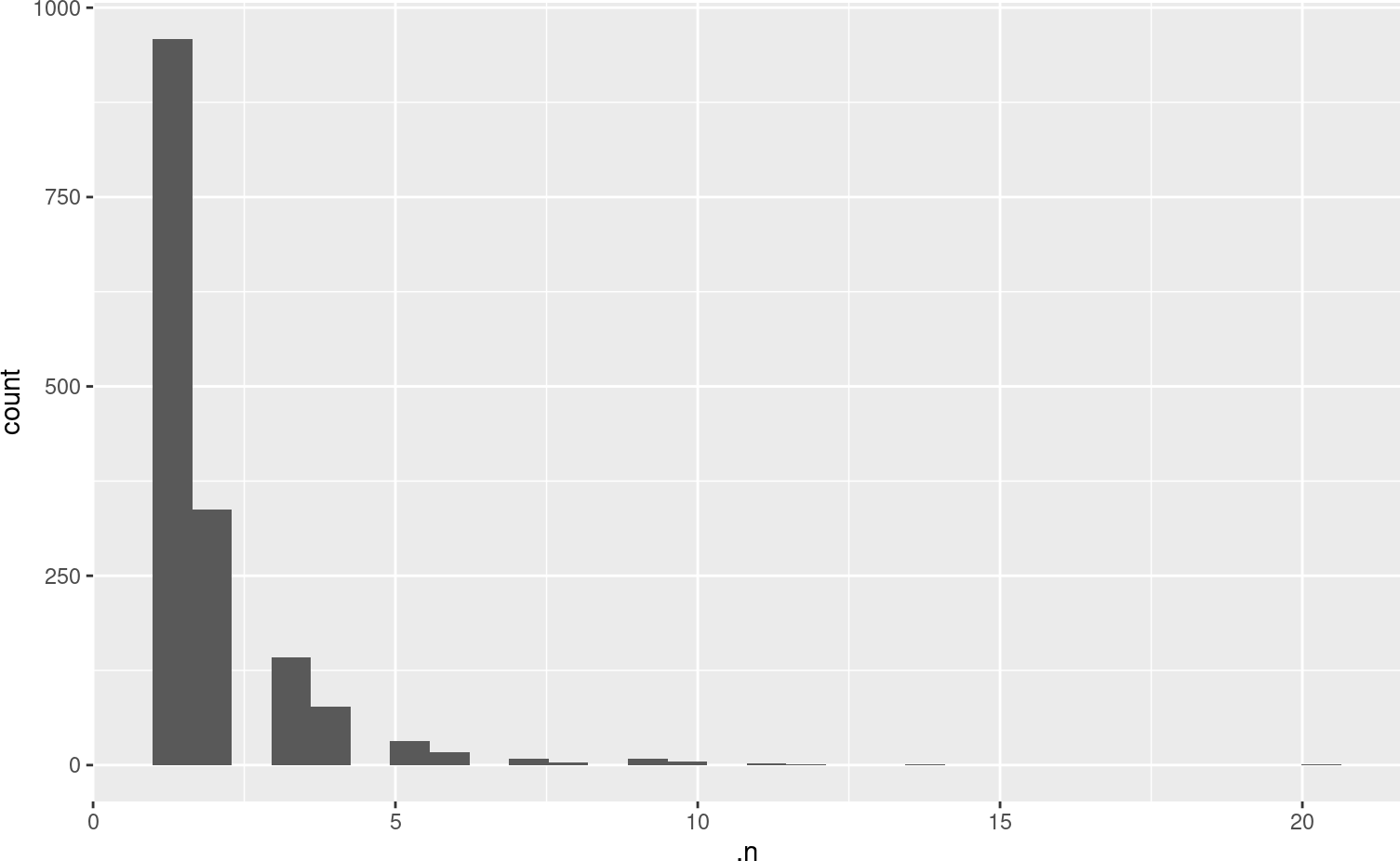We refer the reader to the `ggplot2` package website for more information about the wide variety of functions that the package offers and for tutorials and cheatsheets.

Another useful package for quantitative data exploration is `ComplexHeatmap` (Gu, Eils, and Schlesner (2016)). It is part of the Bioconductor project (Gentleman et al. (2004)) and facilitates visualization of matrix objects as heatmap. See here an example where we plot the protein data.

``````library(ComplexHeatmap)
Heatmap(matrix = assay(hl, "proteins"),
show_row_names = FALSE)``````